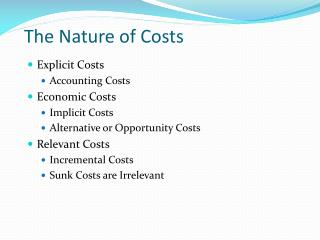DownloadDownload PresentationThe Nature of Costs

# The Nature of Costs

Download Presentation## The Nature of Costs

- - - - - - - - - - - - - - - - - - - - - - - - - - - E N D - - - - - - - - - - - - - - - - - - - - - - - - - - -
##### Presentation Transcript

1. The Nature of Costs • Explicit Costs • Accounting Costs • Economic Costs • Implicit Costs • Alternative or Opportunity Costs • Relevant Costs • Incremental Costs • Sunk Costs are Irrelevant

2. Short-Run Cost Functions Total Cost = TC = f(Q) Total Fixed Cost = TFC Total Variable Cost = TVC TC = TFC + TVC

3. Short-Run Cost Functions Average Total Cost = ATC = TC/Q Average Fixed Cost = AFC = TFC/Q Average Variable Cost = AVC = TVC/Q ATC = AFC + AVC Marginal Cost = TC/Q = TVC/Q

4. Short-Run Cost Functions

5. Short-Run Cost Functions Average Variable Cost AVC = TVC/Q = w/APL Marginal Cost TC/Q = TVC/Q = w/MPL

6. Long-Run Cost Curves Long-Run Total Cost = LTC = f(Q) Long-Run Average Cost = LAC = LTC/Q Long-Run Marginal Cost = LMC = LTC/Q

7. Derivation of Long-Run Cost Curves

8. Possible Shapes ofthe LAC Curve

9. Learning Curves Average Cost of Unit Q = C = aQb Estimation Form: log C = log a + b Log Q

10. Minimizing Costs Internationally • Foreign Sourcing of Inputs • New International Economies of Scale • Immigration of Skilled Labor • Brain Drain

11. Cost-Volume-Profit Analysis Total Revenue = TR = (P)(Q) Total Cost = TC = TFC + (AVC)(Q) Breakeven Volume TR = TC (P)(Q) = TFC + (AVC)(Q) QBE = TFC/(P - AVC)

12. Cost-Volume-Profit Analysis P = 40 TFC = 200 AVC = 5 QBE = 40

13. Operating Leverage Operating Leverage = TFC/TVC Degree of Operating Leverage = DOL

14. Operating Leverage TC’ has a higher DOL than TC and therefore a higher QBE